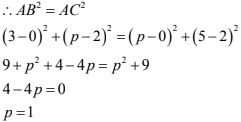# Chapter 14 Co-Ordinate Geometry RD Sharma Solutions Exercise 14.2 Class 10 Maths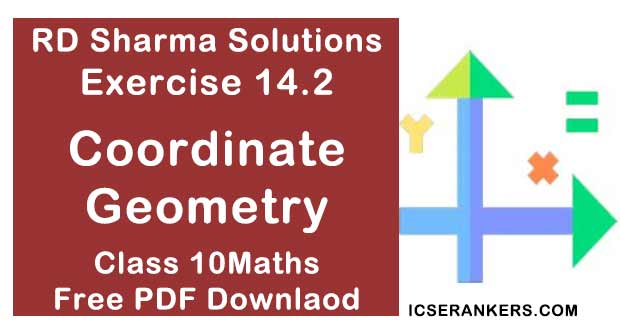Chapter Name RD Sharma Chapter 14 Co-Ordinate Geometry Book Name RD Sharma Mathematics for Class 10 Other Exercises Exercise 14.1Exercise 14.3Exercise 14.4Exercise 14.5 Related Study NCERT Solutions for Class 10 Maths

### Exercise 14.2 Solutions

1. Find the distance between the following pair of points:
(i) (-6, 7) and (-1, -5)
(ii) (a + b, b + c) and (a - b, c -b)
(iii) (a sin α, -b cos α)  and (-a cos α, b sin α)
(iv) (a, 0) and (0, b)

Solution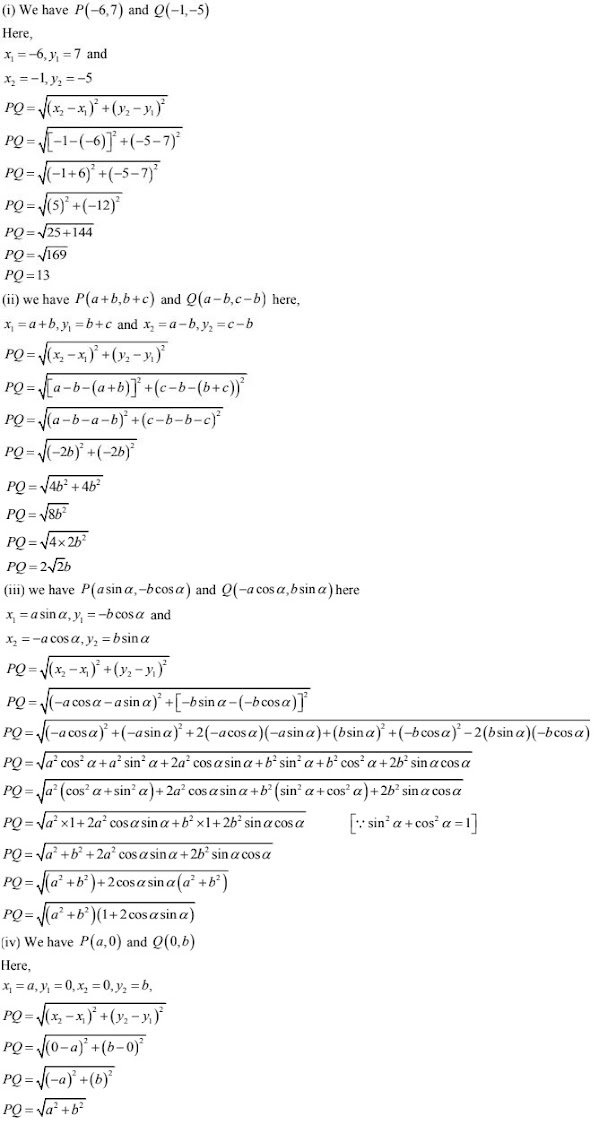2.  Find the value of a when the distance between the points (3, a) and (4, 1) is √10.

Solution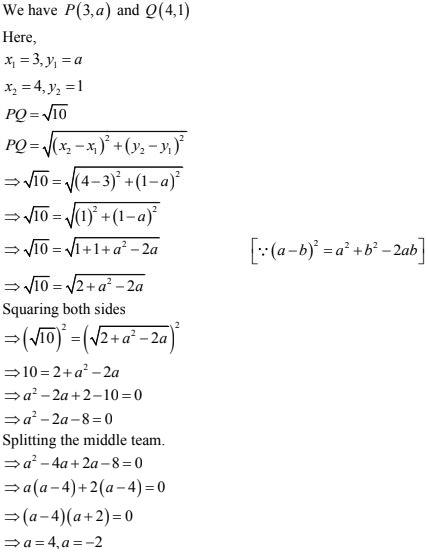3. If the points (2, 1) and (1, -2) are equidistant from the point (x, y) from (-3, 0) as well as from (3, 0) are 4.

Solution

We have P(2, 1) and Q(1, -2) and R(X, Y)
Also, PR = QR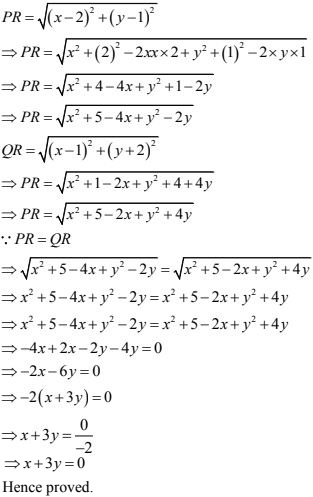4. Find the values of x, y if the distances of the point (x, y)  from (-3, 0) as well as from (3, 0) are 4.

Solution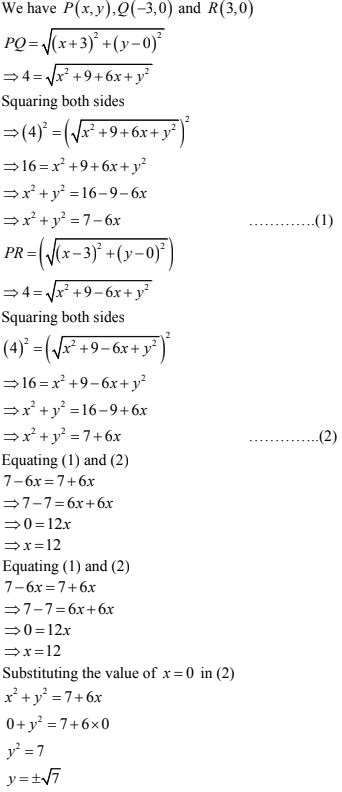5. The length of a line segment is of 10 units and the coordinates of one end - point are (2, -3). If the abscissa of the other end is 10, find the ordinate of the other end.
Solution
Let two ordinate of the other end R be Y
∴ Coordinates of other end R are (10, y) i.e., R(10, y)
Distance PR = 10  [given]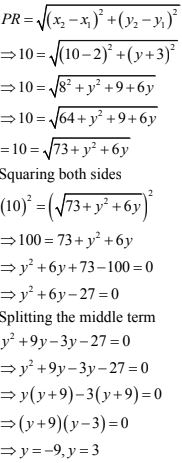6. Show that the points (-2, -4), (4, 0) and (2, 3) are the vertices points of are the vertices points of a rectangle.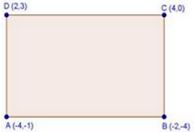Solution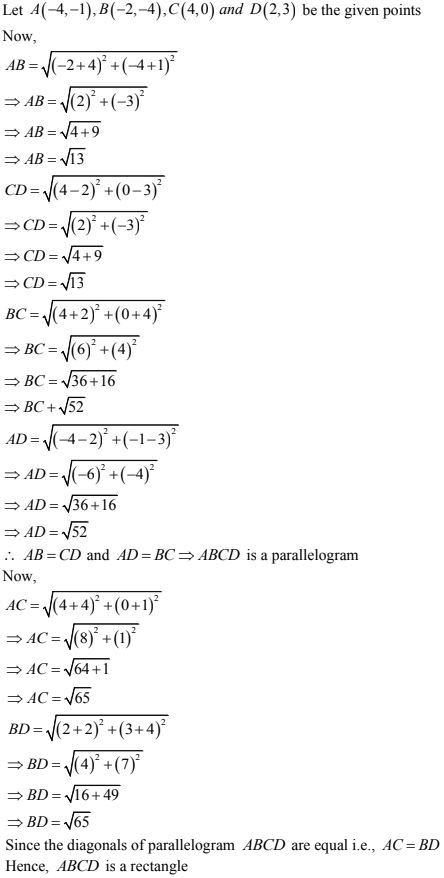7. Show that the points A(1, -2), B(3, 6), C(5, 10) and D(3, 2)  are the vertices of a parallelogram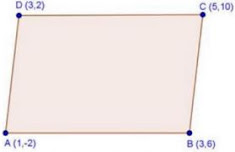Solution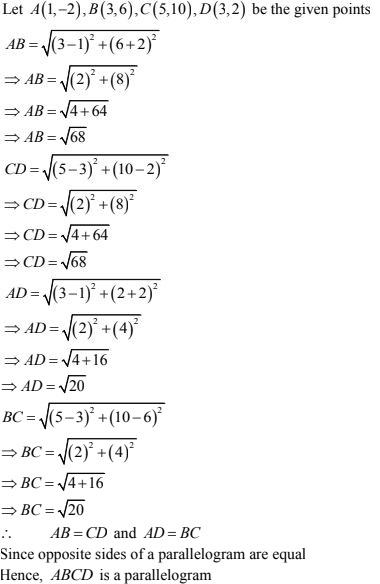8. Prove that the points A(1, 7), B(4, 2), C(-1, -1)  and D(-4, 4) are the vertices of a square.
Solution
Let A(1, 7), B(4, 2), C(-1, -1) and D(-4, 4) be the given point. One way of showing that ABCD is a square is to use the property that all its sides should be equal and both its diagonals should also be equal
Now,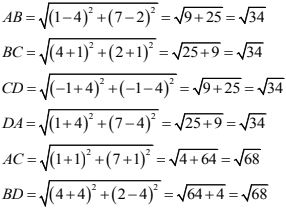Since, AB = BC = CD = DA and AC= BD, all the four sides of the quadrilateral ABCD are equal and its diagonals AC and BD are also equal. Therefore, ABCD is a square.

9. Prove that the points (3, 0) (6, 4) and (-1,3) are vertices of a right angled isosceles triangle.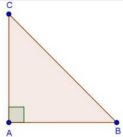Solution
Let A(3,0), B(6, 4) and C(-1, 3) be the given points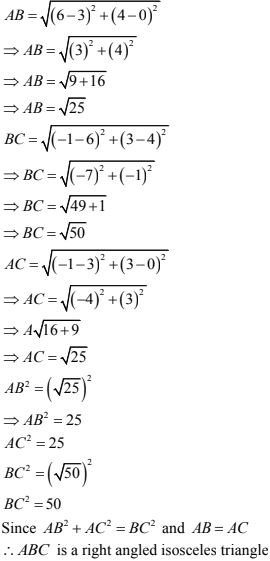10. Prove that (2, - 2), (-2, 1)  and (5, 2) are the vertices of a right angled triangle. Find the area of the triangle and the length of the hypotenuse.
Solution
Let A(2, -2), B(-2, 1) and C(5, 2) be the given points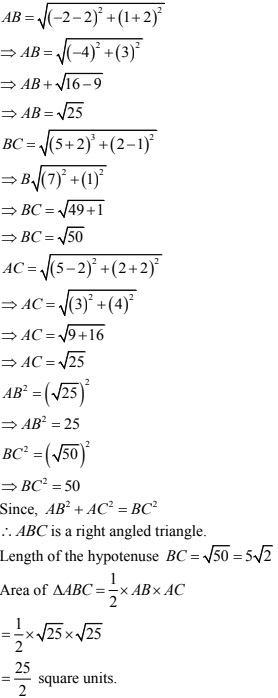11. Prove that the points (2a, 4a) , (2a, 6a) arid (2a + √3a, 5a) be the vertices of an equilateral triangle.
Solution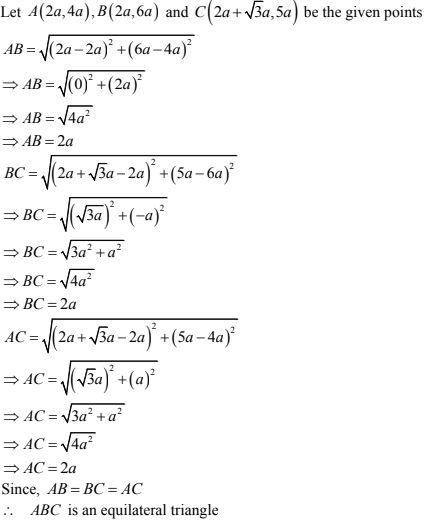12. Prove that the points (2, 3), (-4, -6) and (1,3/2) do not form a triangle.
Solution
Let A(2, 3), B(-4, -6) and C(1, 3/2) be the given points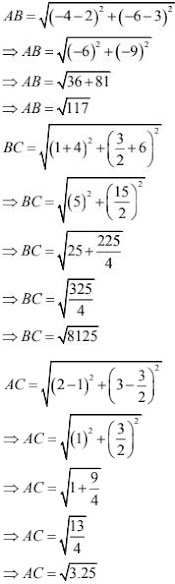We know that for a triangle sum of two sides is greater than the third side. Here AC + BC is not greater than AB.
∴ ABC is not triangle

13. An equilateral triangle has two vertices are (2, -1), (3, 4), (-2, 3) and (-3, -2) find the coordinates of the third vertex.
Solution
Let A(3, 4), B(-2, 3) and C(x, y) be the three vertices of the equilateral triangle then,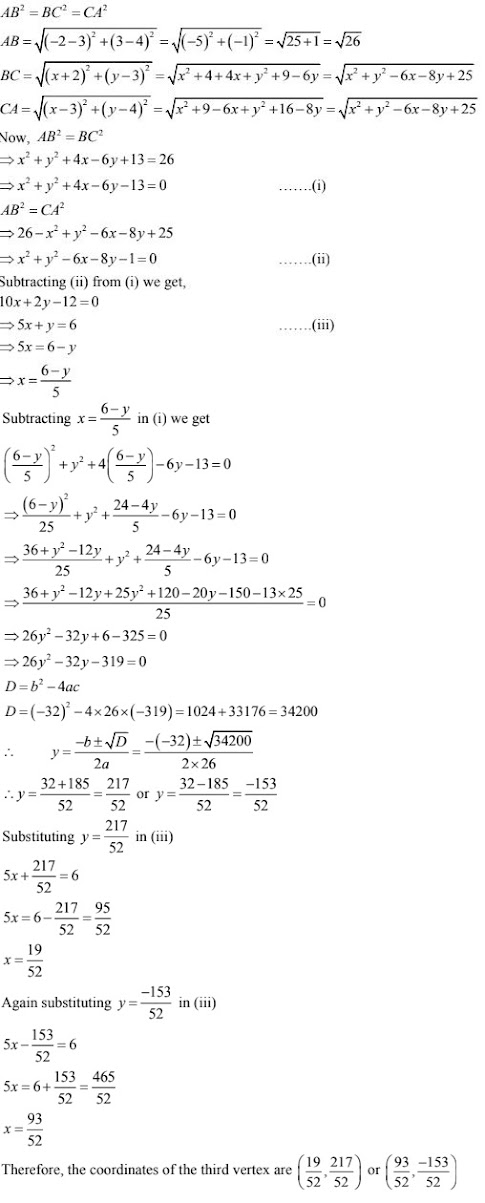14. Show that the quadrilateral whose vertices are (2, -1) (3, 4), (-2, 3) and (-3, -2) is a rhombus.
Solution
Let A(2, -1), B(3, 4), C(-2, 3) and D(-3, -2)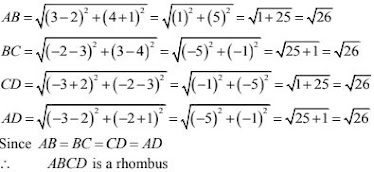15. Two vertices of an isosceles triangle are (2, 0) and (2, 5). Find the third vertex if the length of the equal side is 3.
Solution
Two vertices of an isosceles triangle are A(2, 0) and B(2, 5). Let C(x, y) be the third vertex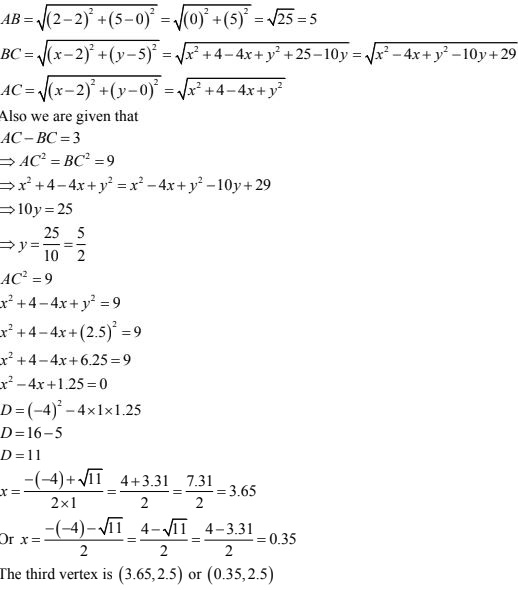16. Which point on x-axis is equidistant from (5, 9) and (-4, 6)?
Solution
Let A(5, 9) and B(-4, 6) be the given points.
Let C(x, 0) be the point on x - axis
Now,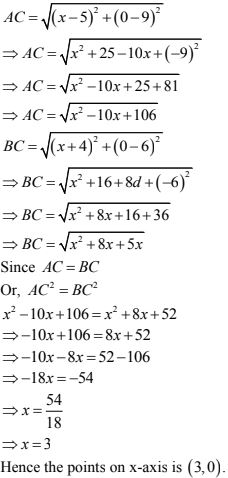17. Prove that the points(-2, 5), (0, 1) and (2, -3) are collinear.
Solution
Let A (-2,5), B(0, 1) and C(2, - 3) be the given points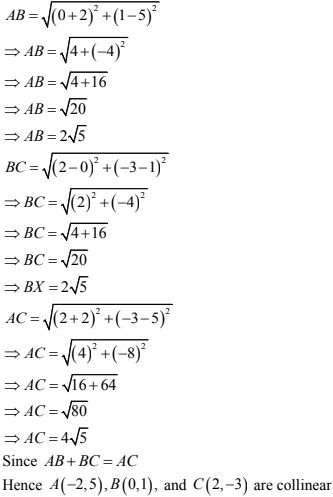18. The coordinates of the point P are (-3, 2). Find the coordinates of the point Q which lies on the line joining P and origin such that OP = OQ.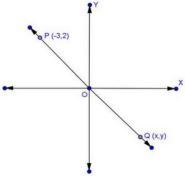Solution
Let the coordinates of Q be (x,y)
Since Q lies on the line joining P and O (origin) and OP = OQ
By mid - point theorem
(x -3)/2 = 0 and (y + 2)/2 = 0
∴ x = 3, y = -2
Hence coordinates of points Q are (3, -2)

19. Which point on y - axis is equidistant from (2, 3) and (-4, 1) ?
Solution
A(2, 3) and B(-4, 1) are the given points.
Let C(0, y) be the points are y - axis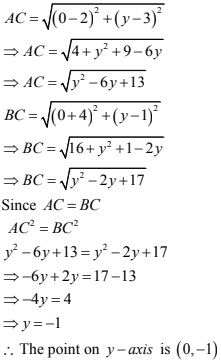20. The three vertices of a parallelogram are (3, 4), (3, 8) and (9, 8).  Find the fourth vertex.
Solution
Let A(3, 4), B(3, 8) and C(9, 8) be the given points
Let the forth vertex be D(x, y)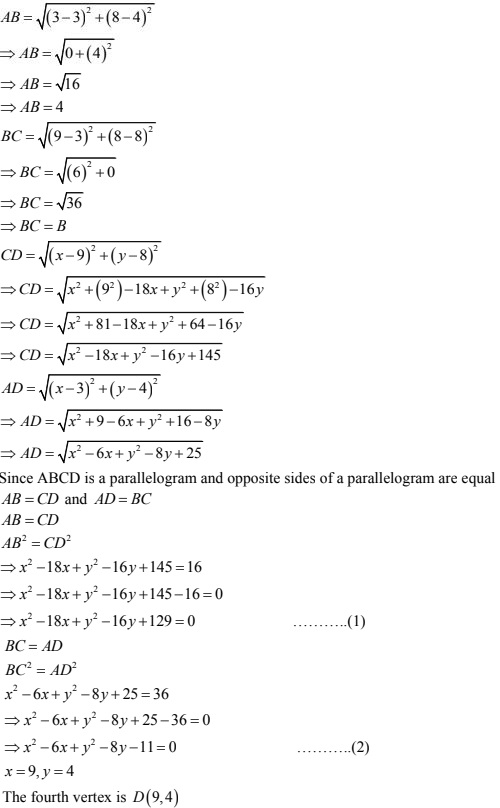21. Find the circumcenter of the triangle whose vertices are (-2, -3), (-1, 0), (7, -6).
Solution
Circumcenter of a triangle is the point of intersection of all the three perpendicular bisectors of the sides of triangle. So, the vertices of the triangle lie on the circumference of the circle.
Let the coordinates of the circumcenter of the triangle be (x, y)
∴ (x, y)  will the equidistant from the vertices of the triangle.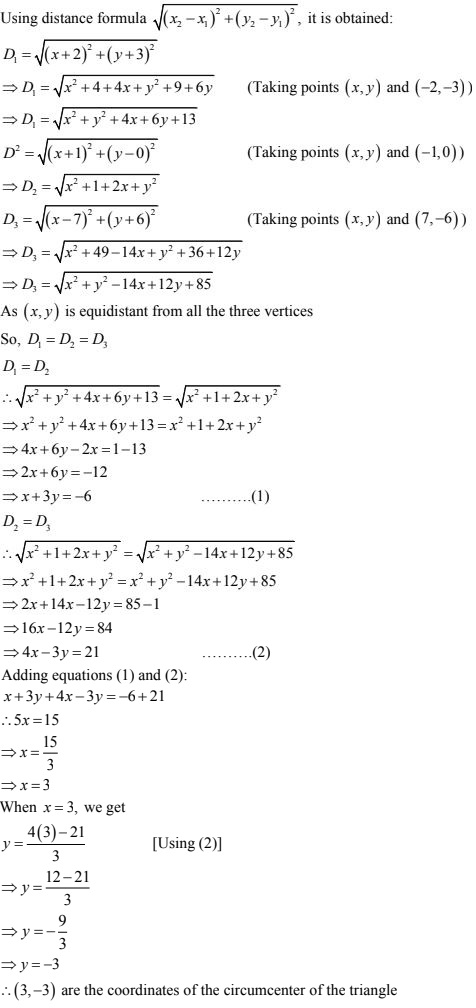22. Find the angle subtended at the origin by the line segment whose end points are (0, 100) and (10, 0).
SolutionLet the point P(0, 100) and Q(10, 0) be the given points.
∴ The angle subtended by the line segment PQ at the origin O is 90° .

23. Find the centre of the circle passing through(5, -8), (2, -9) and (2, 1).
Solution
Let the center of the circle be O (x, y)
Since radii of the circle is constant
Hence, distance of O from A(5, -8), B(2, -9) and C(2, 1) will be constant and equal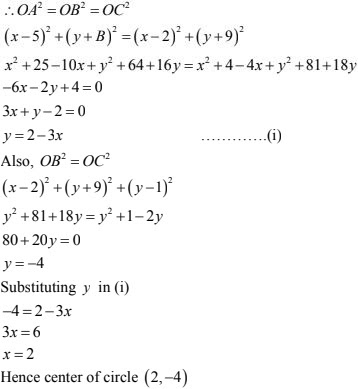24. Find the value of k, if the point P(0, 2) is equidistant from (3, k) and (k, 5).
Solution
Let the point P(0, 2) is equidistant from A(3, k) and (k, 5)
PA = PB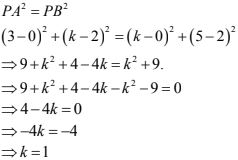25. If two opposite vertices of a square are (5, 4) and (1, -6), find the coordinates of its remaining two vertices.
Solution
Let ABCD be a square and let A(5, 4)  and C(1, -6) be the given points.
Let (x, y)  be the coordinates of B.
AB = BC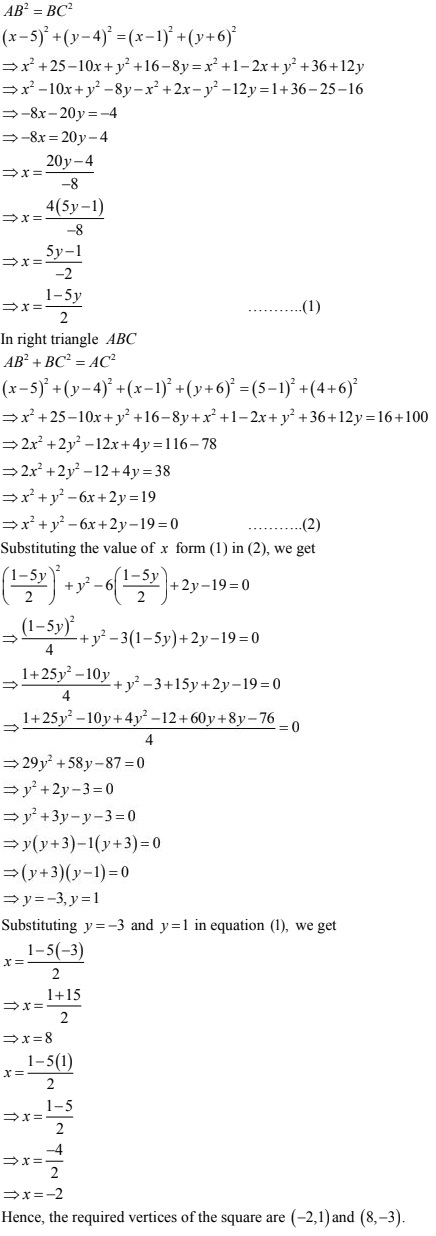26. Show that the points(-3, 2), (-5, -5), (2, -3) and (4, 4) are the vertices of a rhombus.
Find the area of this rhombus.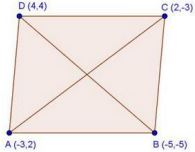Solution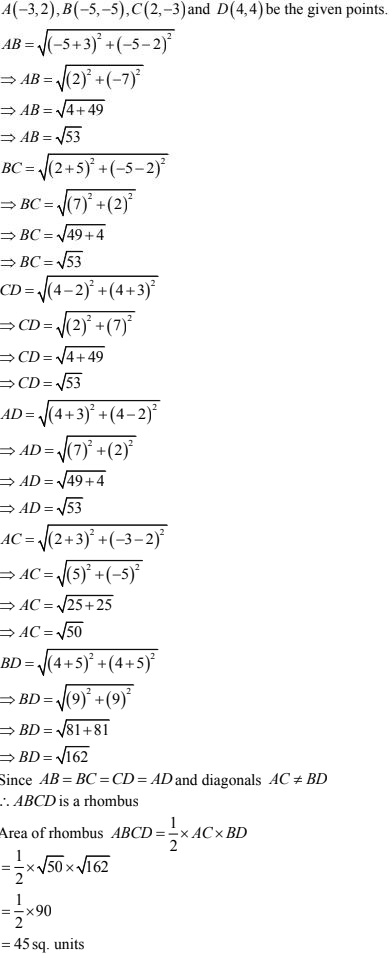27. Find the coordinates of the circumcenter of the triangle whose vertices are (3, 0), (-1, -6) and (4, -1). Also, find its circumradius.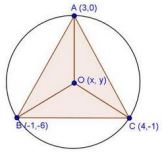Solution
Let A(3, 0), B(-1, -6) and C(4, -1) be the given points.
Let O(x, y) be the circumcenter of the triangle
OA = OB = OC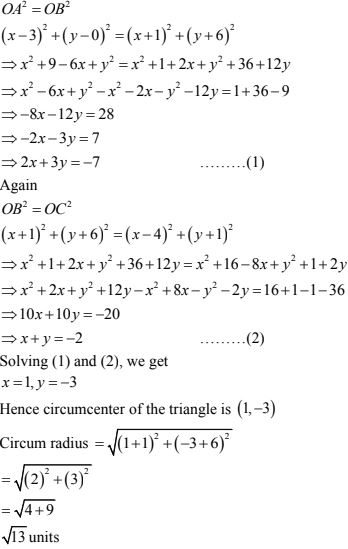28. Find a point on the x - axis which is equidistant from the points (7, 6) and (-3, 4).
Solution
Let A(7, 6) and B(-3, 4) be the given points.
Let P(x, 0) be the point on x - axis such that PA = PB
PA = PB29. (i) Show that the points A(5, 6), B(1, 5) C(2, 1) and D(6, 2) are the vertices of a square.
(ii) Prove that the points A(2, 3), B(-2, 2), C(-1, -2) and D(3, -1) are the vertices of a square ABCD.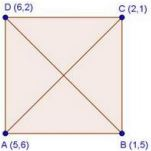Solution
A(5, 6), B(1, 5), C(2, 1) and D(6, 2) are the given points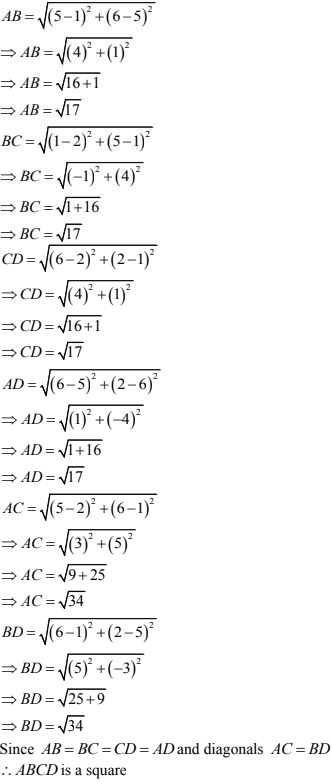30. Find the point on x-axis which is equidistant from the points (-2, 5) and (2, -3).
Solution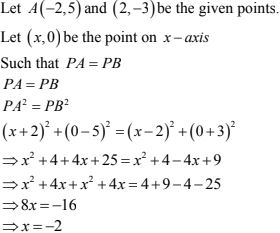31. Find the value of x such that PQ = QR where the coordinates of P, Q and R are (6,—1), (1,
3) and (x, 8) respectively.
Solution
P(6, -1), Q(1, 3) and R(x, 8) are the given points.
PQ = QR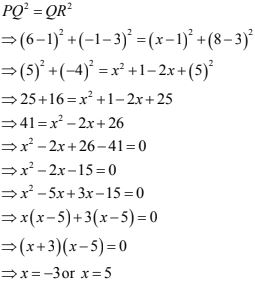32. Prove that the points (0, 0), (5, 5) and (-5, 5) are the vertices of a right isosceles triangle .
Solution
Let A(0, 0), B(5, 5) and C(-5, 5) be the given points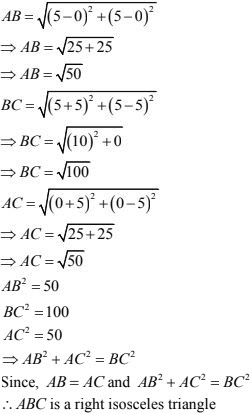33. If the point P(x, y ) is equidistant from the points A(5, 1) and B (1, 5), prove that x = y.
Solution
Since P(x, y) is equidistant from A(5, 1) and B(1, 5)
AP = BP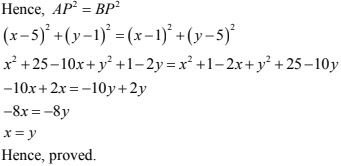34. If Q (0, 1) is equidistant from P (5, —3) and R (x, 6), find the values of x. Also, find the
distances QR and PR
Solution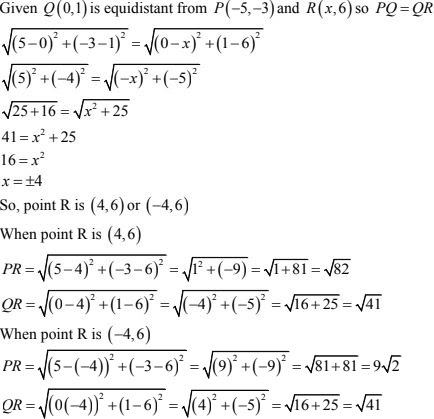35. Find the values of y for which the distance between the points P (2, —3) and Q (10, y) is
10 units
Solution
Given that distance between (2, -3) and (10, y) is 10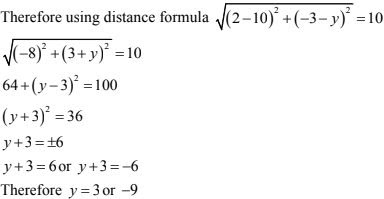36. Find the centre of the circle passing through (6, -6), (3, -7) and (3, 3)
Solution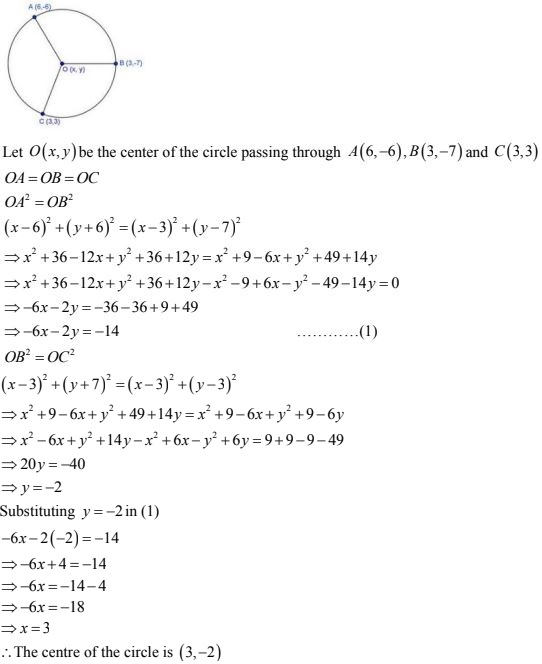37. Two opposite vertices of a square are (-1, 2) and (3, 2). Find the coordinates of other two vertices.
Solution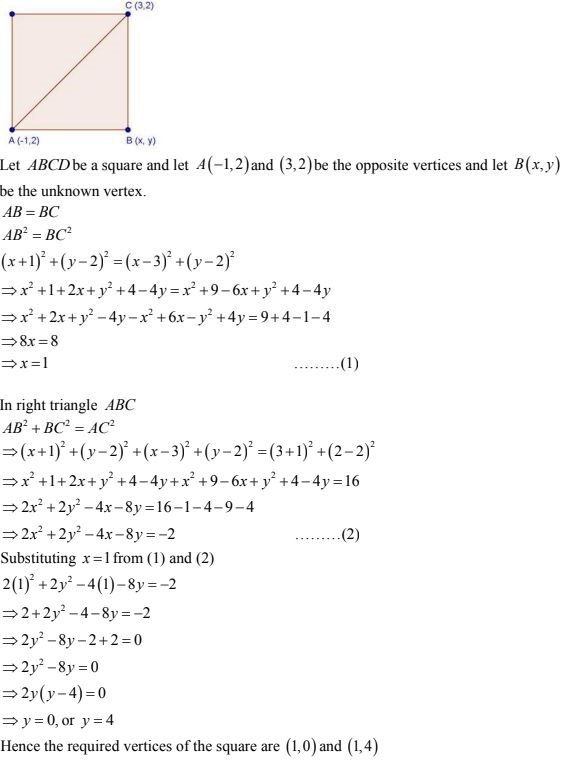38. Name the quadrilateral formed, if any, by the following points, and give reasons for your answers:
(i) A(-1, -2), B(1, 0), C(-1, 2), D(-3, 0)
(ii) A(-3, 5), B(3, 1), C(0, 3), D(-1, -4)
(iii) A(4, 5), B(7, 6), C(4, 3), D(1, 2)
Solution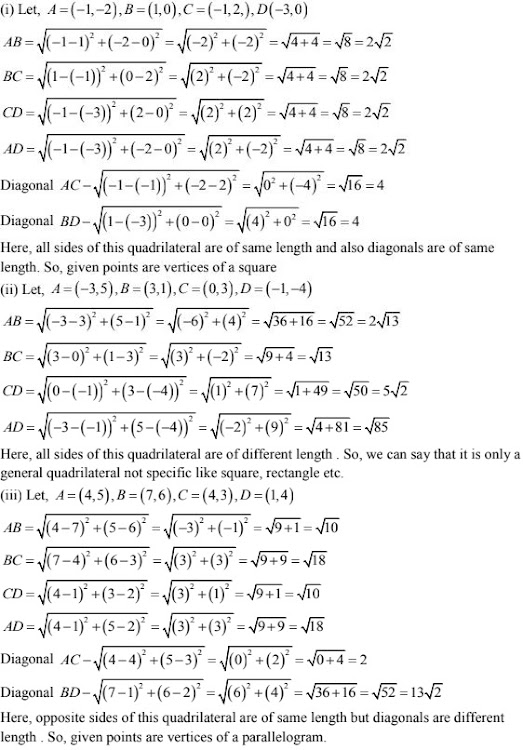39. Find the equation of the perpendicular bisector of the line segment joining points (7, 1) and (3, 5).
Solution
Bisector passes through midpoint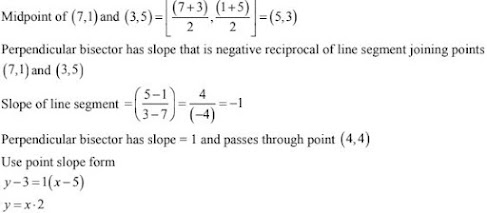40. Prove that the points (3, 0), (4, 5), (—1, 4) and (—2, —1), taken in order, form a rhombus.
Also, find its area.
Solution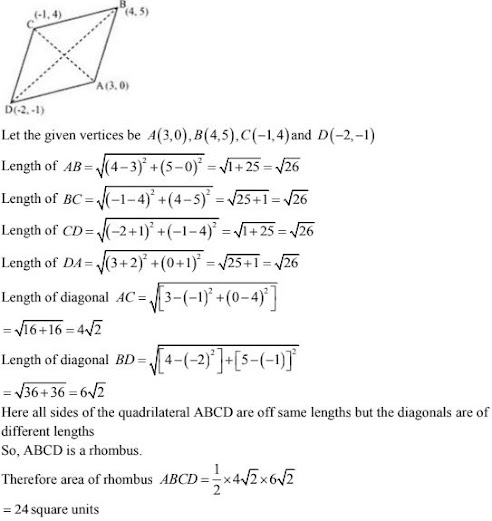41. In the seating arrangement of desks in a classroom three students Rohini, Sandhya and Bina are seated at A (3, 1), B (6, 4) and C (8, 6). Do you think they are seated in a line?
Solution
Let A(3, 1), B(6, 4) and C(8, 6) be thee given points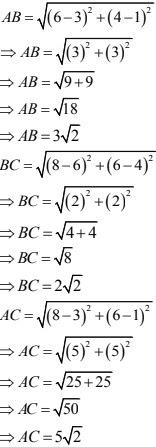Since, AB + BC = AC
Points A, B, C are collinear
Hence, Rohini, Sandhya and Bina are seated in a line

42. Find a point on y - axis which is equidistant from the points (5, -2) and (-3, 2).
Solution
Let A(5, -2) and B(-3, 2) be the given points,
Let P(0, y) be the point on y - axis
PA = PB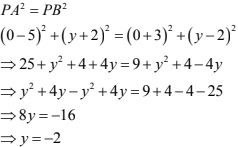43. Find a relation between x and y such that the point (x, y) is equidistant from the points (3, 6) and (-3, 4).
Solution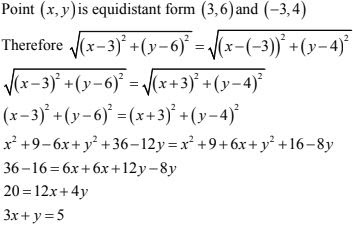44. If a point A(0, 2) is equidistant from the point B(3, p) and C(p, 5), then find the value of p.
Solution
A(0, 2), B(3, P) and C(p, 5) are given points
It is given that AB = AC